Next: Bibliography Up: PhD Thesis Heinrich Kirchauer Previous: C.2 Stack of Homogeneous

# D. Nonplanar Material Interface

The error introduced by the truncation (6.27) of the Fourier expansion of the EM field is now investigated for the stepped topography of Figure 8.16. A sketch of the relevant region of the geometry is re-plotted in Figure D.1. All derivations are performed in two dimensions only since the results apply to three dimensions as well.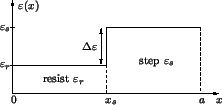The lateral dependence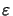(x) of the permittivity is simply given by (cf. Figure D.1)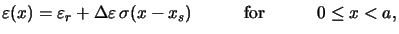(D.1)

with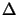=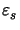-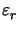as the difference of the resist and step material permittivities. The intersection of the cut-line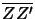with the step slope is denoted by xs, and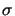(x) refers to Heaviside's step function.a The differential method requires the Fourier coefficients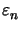of the permittivity function(x) of (D.1),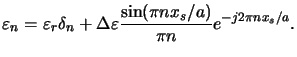(D.2)

We restrict our investigations to TE-polarized light. In this case the Fourier coefficients Ey, n(z) of the y-component of the electric field satisfyb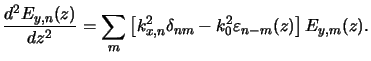(D.3)

The impact of the truncation of the Fourier series can best be understood by explicitly studying one specific mode Ey, i(z) at an arbitrary vertical cut-position zc, e.g., atas indicated in Figure D.1. For the analysis the amplitude of Ey, i(zc) can be chosen arbitrarily. We conveniently set it equal to 1/k02 so that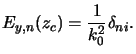(D.4)

Inserting this relation into (D.3) gives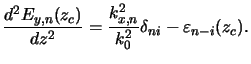(D.5)

For simplicity we consider only the case when kx, n2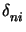vanishes, i.e., the propagation of the vertical mode i = 0 or, equivalently, the energy spread of an arbitrary order i into the zeroth-order n = 0 is studied. Since the vertical propagating mode usually carries most of the energy, this restriction gives still valuable information.

The impact of the truncation of the Fourier expansions (6.27) is now quantified by the following measure describing the ratio between lost'' and total'' energy spread: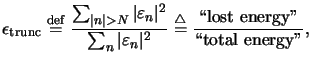(D.6)

This quantity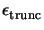can be interpreted as truncation error since it describes the error made in the calculation of the second derivative (cf. (D.5)). Note that the measure is restricted to n = 0 or i = 0 since only then the first term kx, nvanishes in (D.5). The extension to the general situation is self-evident.

We will now derive an upper bound for. For that we first evaluate the denominator occurring in (D.6) with the help of Parseval's theorem [131, p. 224] to (cf. (D.1))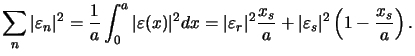(D.7)

Next we consider the numerator of (D.6). An upper bound for N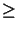1 is given by (cf. (D.2))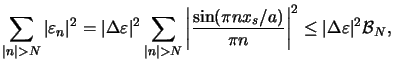(D.8)

with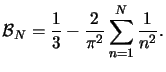(D.9)

The bound is obtained by replacing | sin(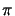nxs/a)| with unity, i.e.,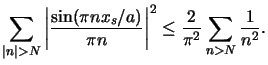(D.10)

The right-hand side equals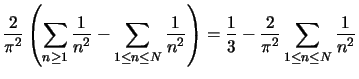(D.11)

since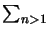n-2 =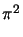/6. Inserting (D.7) and (D.8) into (D.6) yields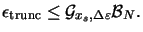(D.12)

with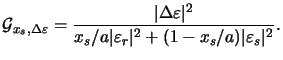(D.13)

(D.12) shows that the bound consists of a geometrical factor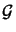xs,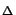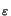and a numerical factor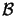N. The geometrical factorxs,(cf. (D.13)) is proportional to the squared difference ||2 = |-|2 of the resist and step material permittivities. The truncation erroris thus governed by the strength of the inhomogeneity, which is in accordance with physical considerations. The dependence of the numerical factorN (cf. (D.9)) on the truncation frequency N is plotted for a constantxs,in Figure D.2. In this figure also an exact expression for the special situation xs = a/2 is shown, i.e., the case when the intersection point xs lies in the middle of the interval [0, a]. In this case the truncation errorof (D.6) can be exactly calculated like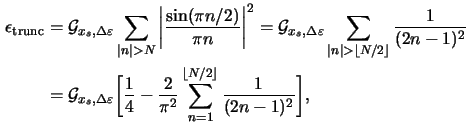whereby(2n - 1)-2 =/8 is used. Both expressions (D.12) and (D.14) show that for increasing N the error decreases quadratically.

Figure D.2: Truncation errorof the second derivative (cf. (D.6)) as a function of the cut-off frequency N. The geometrical factor is set equal to unity. Both the bound (D.12) and the exact expression (D.14) obtained for xs = a/2 are shown in linear and logarithmic scale, upper and lower graph, respectively. For increasing N the error decreases quadratically.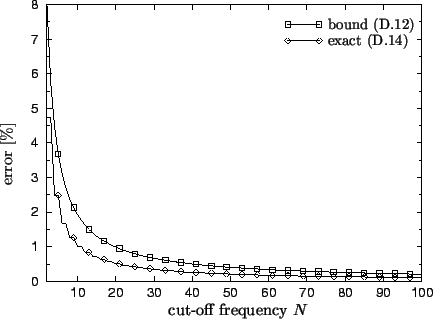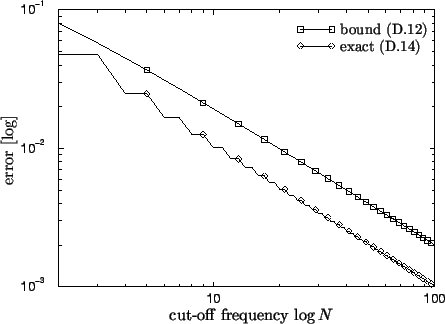As a final remark note that (D.6) describes the error of the second derivative at one single vertical point zc. The overall discretization error depends on the number Nz of vertical grid points.

#### Footnotes

... function.a
Heaviside's step function is defined as(x) = 0 for x < 0 and 1 otherwise.
... satisfyb
For TE-polarization the three field components Ex(z), Ez(z), and Hy, nm(z) vanish [11, p. 52]. Taking the remaining three field components Ey(z), Hx(z), and Hz(z) in (6.15) and (6.18) and eliminating the latter two yields (D.3).Next: Bibliography Up: PhD Thesis Heinrich Kirchauer Previous: C.2 Stack of Homogeneous
Heinrich Kirchauer, Institute for Microelectronics, TU Vienna
1998-04-17